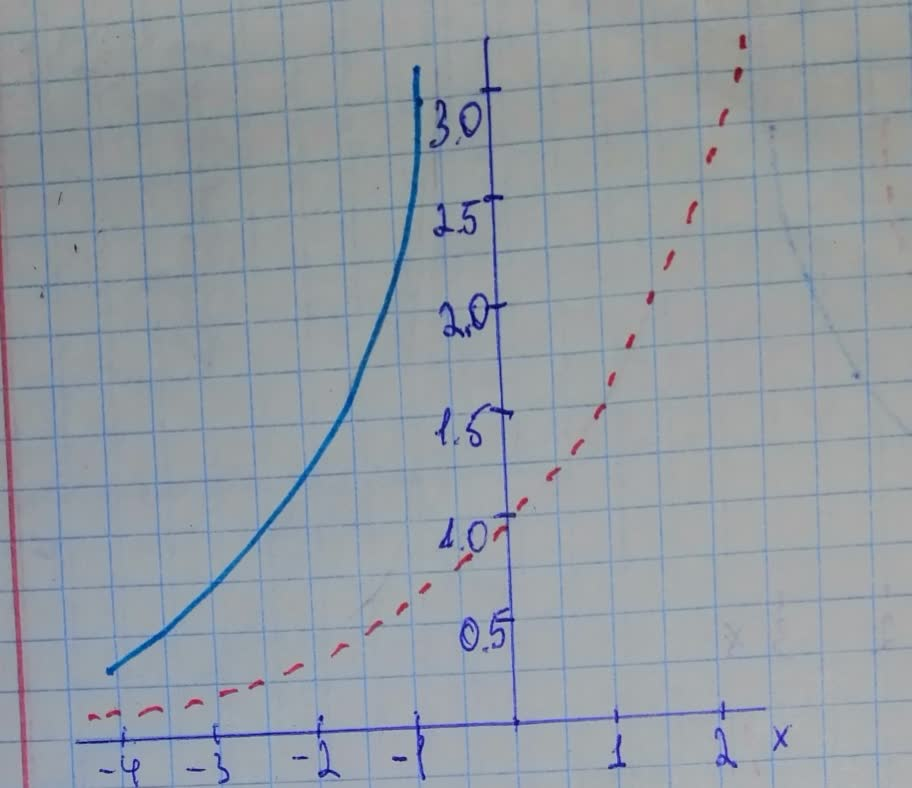#### Didn’t find what you are looking for?

Question# Begin by graphing f(x)=2^{x}. Then use transformations of this graph to graph the given function. Be sure to graph and give equations of the asymptote

Transformations of functions
ANSWEREDBegin by graphing
$$\displaystyle{f{{\left({x}\right)}}}={2}^{{{x}}}.$$
Then use transformations of this graph to graph the given function. Be sure to graph and give equations of the asymptotes. Use the graphs to determine each function's domain and range. If applicable, use a graphing utility to confirm your hand-drawn graphs.
$$\displaystyle{g{{\left({x}\right)}}}={2}^{{{x}\ +\ {2}}}$$2020-12-25
Step 1Step 2
Graph of $$\displaystyle{2}^{{{x}}}$$ is shown as a dotted curve.
The graph of $$\displaystyle{2}^{{{x}\ +\ {2}}}$$ is the graph of $$\displaystyle{2}^{{{x}}}$$ translated left by two units.
Its asymplote has equation $$\displaystyle{y}={0}$$